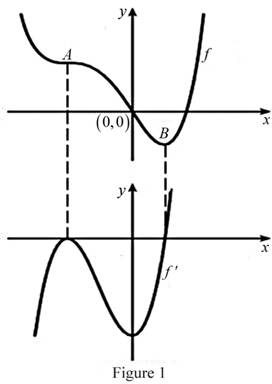# The graph of f ′ below the graph of f.### Single Variable Calculus: Concepts...

4th Edition
James Stewart
Publisher: Cengage Learning
ISBN: 9781337687805### Single Variable Calculus: Concepts...

4th Edition
James Stewart
Publisher: Cengage Learning
ISBN: 9781337687805

#### Solutions

Chapter 2.7, Problem 10E
To determine

## To sketch: The graph of f′ below the graph of f.

Expert Solution

### Explanation of Solution

From the given graph, it is observed that the graph of f contains the horizontal tangents at two points. Let the two points be A and B.

Note that, the value of the derivative will be zero at the point where the function has the horizontal tangent.

Thus, the graph of f will be zero at the points A and B.

From the point A to left, the graph has strictly negative slope which implies that the derivative graph f must have a functional value in negative value.

From the point B to right, the graph has strictly positive slope which implies that the derivative graph f must have a functional value in positive.

Graph:

Use the above information and trace the graph of f(x) as shown below in Figure 1.Thus, f is the required graph.

### Have a homework question?

Subscribe to bartleby learn! Ask subject matter experts 30 homework questions each month. Plus, you’ll have access to millions of step-by-step textbook answers!